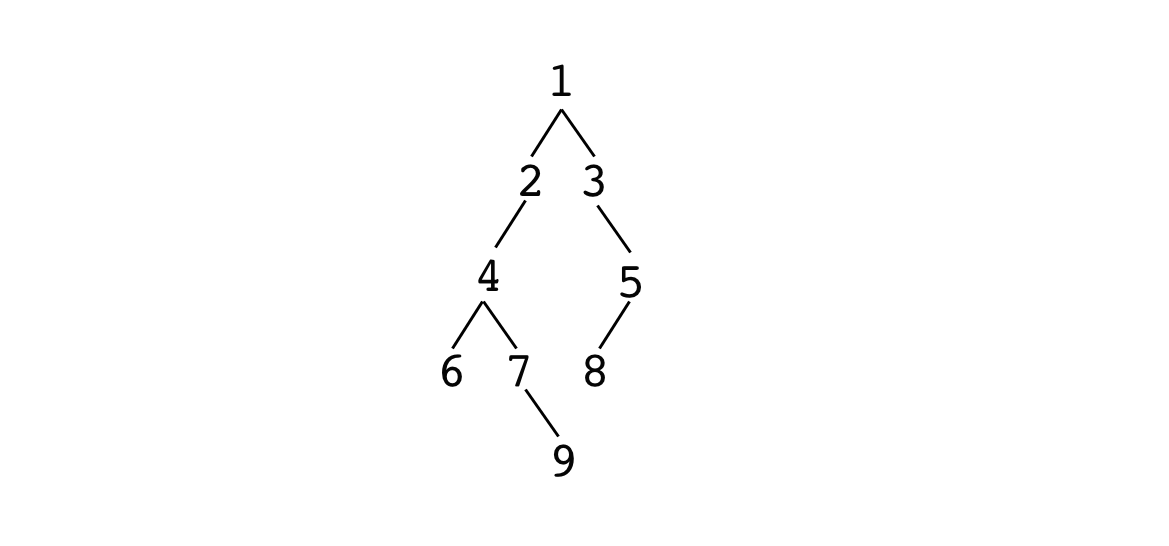# 110. serialize and deserialize binary tree

JavaScriptAlgorithm

medium  - accepted / - tried

Can you transform(serialize) a binary tree into a string and restore(deserialize) a binary tree from the string? Just like what JSON.stringify() and JSON.parse() do.

For example, for a tree from 91. invert a binary treeBFE.dev would serialize it to `[1,2,3,4,null,null,5,6,7,8,null,null,null,null,9]`

But there are more ways of doing it rather than above, any would be fine as long as your `deserialize()` and `serialize()` work as a pair.

Your code is tested like this:

``````
const tree1 = ...
expect(typeof serialize(tree1)).toBe('string')

const tree2 = deserialize(serialize(tree1))
expect(isIdentical(tree1, tree2)).toBe(true)``````

Binary tree in this problem consists of value of integers.

Bugfree ordinary solution is better than buggy fancy ones.

(87)